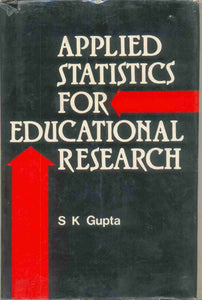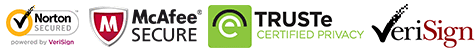# Applied Statistics For Educational Research

Regular price Rs. 190.00

The book is divided into two parts: Part first defines the statistics useful for research with a clarity in concept. Actually the statistical terms have been operationally defined with exactness for the use of the statistical methods in research. In ordinary books on statistics, this part is generally missing. Those books simply focus on the calculation part. If the students do nor understand the computation parts of statistics together. The Part second of the book deals with the advanced statistical methods in Research. Special consideration has been given to the applied part. The statistical methods have been discussed with suitable exemplary problems and details of computations. The topics that have been thoroughly discussed are (i) Normal distribution curve, (ii)) Chi-square statistics, (iii) Non-parametric statistics other than chi-ssquare (iv) Regression and prediction, (v) Factors analysis. The Applied Statistics for Educational Research will serve as a text book for the students of M.A. (Education), M.A. (Psychology), M.Ed. M.Phil. (Education) of Indian Universities. It will also serve as a reference book for Ph.D. students of education and psychology. It covers the syllabi in advanced statistics for Post Graduate classes as well as covers the use of statistical methods for research in Education at the University level.

Guaranteed Safe Checkout